Open in App
Not now

# Methods of Valuation of Goodwill

• Last Updated : 02 Sep, 2022

Generally, Goodwill means a ‘reputation’ or a ‘good name’. Therefore, a Partnership firm’s Goodwill is the reputation earned by the firm through rendering quality services to its customers. A satisfied customer will come back to the firm again and again thereby, helping the firm to build up a solid customer base that yields more profit in the future. Thus, Goodwill is a market value of the reputation of the firm that enables the firm to earn a profit above the normal profit earned by the other firms in the same industry. Goodwill is an intangible asset that cannot be touched or seen but is not a fictitious asset as it has some value in case of profit-making concern.

” The term Goodwill is generally used to denote the benefit arising from connections and reputation.” – Lord Lindley

“Goodwill may be said to be that element arising from the reputation, connections, or other. advantages possessed by a business which enables it to earn greater profits than the return normally to be expected on the capital represented by the net tangible assets employed in the business.” – Spicer and Pegler

### Accounting Treatment of goodwill:

According to AS-26, Goodwill being an intangible asset shall be recorded in the books only when the consideration in money or money’s worth for such asset has been paid off. Therefore,

• When consideration in money or money’s worth for goodwill is raised and recorded in the books, it shall be written off immediately.
• When no consideration in money or money’s worth is paid for the goodwill, such as in the case of Change in Profit-sharing Ratio among the partners, Admission of a New Partner, Retirement, or Death of a Partner, it is not recorded in the books of the firm. Whenever the profit-sharing ratio among the partners is changed, the share of some of the partners is increased and the share of some of the partners is decreased. So, the partner or partners whose share has been increased (gaining partners) shall make compensation to those partners who have sacrificed their share (sacrificing partners). This compensation payable by the gaining partner to the sacrificing partners is called “Goodwill” or “Premium for Goodwill”. The gaining partner shall pay the share of goodwill to the sacrificing partner equal to the share gained by him. Since the goodwill is not recorded in the book of the firm, it is directly adjusted from the Capital/Current account of the partners. The Capital/Current account of the gaining partner is debited, and that of the sacrificing partner is credited by the amount of compensation.

Amount of Compensation Payable = Value of the firm’s goodwill × Share of the Profit Gained

Suppose, A and B are partners sharing profit in a ratio of 3:2. From 1st April 2022, they decided to share the profit equally. For this purpose, the goodwill of the firm has been valued at ₹60,000. Under this circumstance, A has to sacrificeof his share, and B gainsof the share. Therefore, the amount of compensation (goodwill) payable by B to A is ₹6,000 ().

Journal Entries:

When there is a change in Profit-Sharing Ratio, following entries are passed to make adjustments for the Goodwill:

I. In the case of the Fixed Capital Method: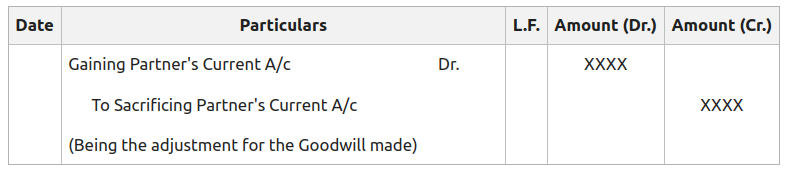II. In the case of the Fluctuating Capital Method: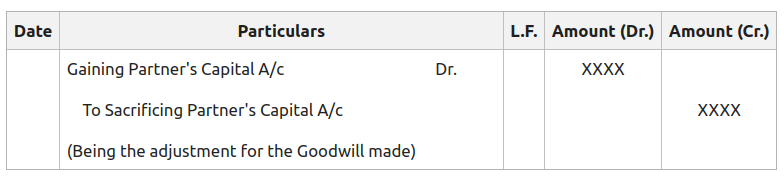Illustration:

Ram and Sham are partners sharing profit in a ratio of 3:2. They decided to share the profit equally in the future. Pass necessary Journal entries to make adjustments for the goodwill when the Goodwill of the firm is valued at ₹ 50,000.

Solution:

Calculation of Gaining ratio and Sacrificing ratio:

Old Ratio of Ram and Sham = 3:2

New Ratio of Ram and Sham = 1:1

Sacrifice or Gain:

Ram =Sham =Amount of Premium of Goodwill (Compensation) payable by Sham to Ram for his gain = 50,000 × 1/10 = ₹5,000.

I. In the case of the Fixed Capital Method: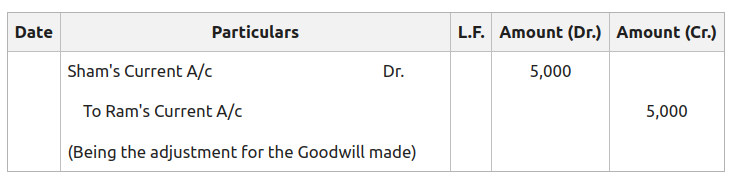II. In the case of the Fluctuating Capital Method: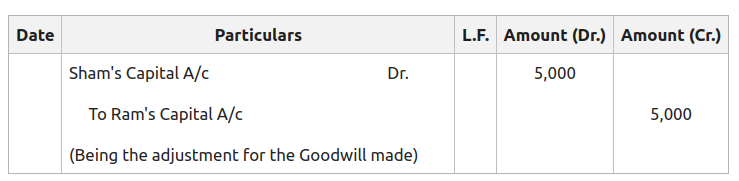### Methods of Valuation of Goodwill:

The Three Methods of Valuing Goodwill have been discussed below:

#### 1. Average Profit Method:

The Average Profit Method is the most simplest and widely used method for the valuation of Goodwill. The following factors are considered under this method:

• The abnormal profit of the year is subtracted from the net profit of that year.
• The abnormal loss of the year is added to the net profit of that year.
• Income not earned from the business operations, such as income from investments is deducted from the net profit of that year.

This method is further divided into two methods:

A. Simple Average Profit Method:

In this method, the number of past years’ profits serves as the basis for the valuation of goodwill. The average of such profits is calculated by dividing the total of such profits by the number of years. Then, the average of the profits is multiplied by the agreed number of years of purchase to calculate the value of Goodwill.

Average Profit =Value of Goodwill = Average Profit × Number of Years of purchase

Illustration:

Calculate the value of the firm’s goodwill on the basis of 2 years of the purchase of the average profit for the last three years.

2019- ₹80,000 (Including an Abnormal gain of ₹ 10,000)

2020- ₹1,00,000 (After charging Abnormal loss of ₹20,000)

2021- ₹90,000

Solution:

Calculating Average Profit: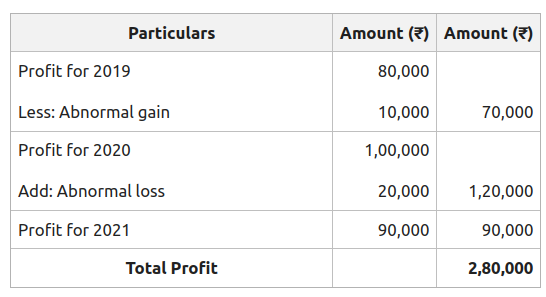Average Profit =Average Profit =Average Profit = 93,334

Calculating Value of Goodwill:

Value of Goodwill = Average Profit X Number of Years of purchase

Value of Goodwill = 93,334 X 2

Value of Goodwill = ₹1,86,668.

B. Weighted Average Profit Method:

The weighted Average Profit Method is a modified form of the Average Profit Method, under which each profit is assigned a weight. More weightage is assigned to the profits of the latest year to ensure the accuracy in the calculation of anticipated future profits and Goodwill. The weighted Average Profit Method is preferable when the profit over the past consecutive years shows a continuous rise or fall.

In this method following, steps are to be followed to calculate the value of the goodwill:

Step 1: Assigning weightage to the profits. The highest weight is assigned to the profit of the most recent year.

Step 2: Product of profits is calculated by multiplying the weight with the respective profit of that particular year.

Step 3: Weighted Average Profit is then calculated by dividing the Total of products of profits by the Total Weights.

Weighted Average Profit = Total of products of profits ÷ Total Weights

Step 4: The value of Goodwill is calculated by multiplying the Weighted Average Profit by the Number of years of purchase.

Goodwill = Weighted Average Profit × Number of years of purchase

Illustration:

Calculate the value of Goodwill based on two years’ purchase of the weighted average profit after assigning weights 1,2,3,4,5 and to the profits for 2018, 2019, 2020, 2021, and 2022, respectively from the following information: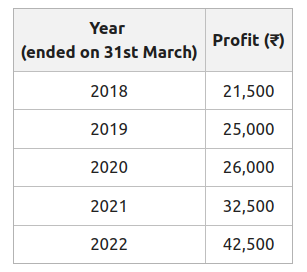Solution:

Calculating Weighted Average Profit: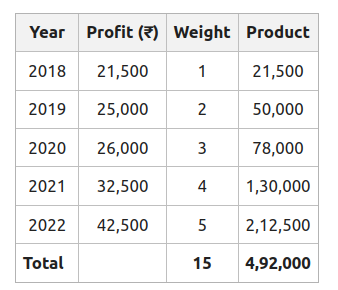Weighted Average Profit =Weighted Average Profit =Weighted Average Profit = ₹32,800

Calculating Value of Goodwill:

Goodwill = Weighted Average Profit X Number of years of purchase

Goodwill = 32,800 X 2

Goodwill = ₹65,600

#### 2. Super Profit Method:

Under Super Profit Method, the value of a firm’s goodwill is calculated on the basis of the Super Profit. Super Profit is the excess profit earned by a firm in comparison to the average profits earned by other firms in the same industry or trade. The firm has no goodwill if there is no anticipated Super Profit.

Steps to calculate the value of Goodwill under the Super Profit Method:

Step 1: Calculation of Actual Average Profit.

Average Profit =Step 2: Calculation of Normal Profit.

Normal Profit =Step 3: Calculation of Super Profit:

Super Profit = Actual or Average Profit − Normal Profit

Step 4: Calculation of Firm’s Goodwill.

Goodwill = Super Profit × Number of Years of Purchase

Illustration:

Calculate the value of Goodwill on the basis of 2.5 years of purchases of average super-profits earned during the mentioned seven years. The Capital invested in the firm is ₹6,00,000, and the Normal rate of return in the same industry is 10%. The net profit for the last seven years are: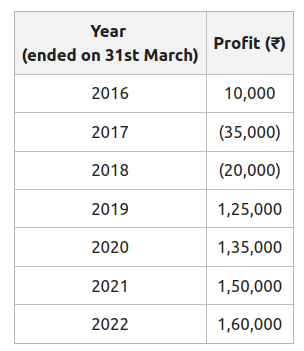Solution:

Calculating Actual Average Profit:

Total of Profits = 10,000 + (35,000) + (20,000) + 1,25,000 + 1,35,000 + 1,50,000 + 1,60,000

Total of Profits = ₹5,25,000

Average Profit =Average Profit =Average Profit = ₹75,000

Calculation of Normal Profit:

Normal Profit =Normal Profit =Normal Profit = ₹ 60,000

Calculation of Super Profit:

Super Profit = Actual or Average Profit − Normal Profit

Super Profit = 75,000 − 60,000

Super Profit = ₹15,000

Calculation of Firm’s Goodwill:

Goodwill = Super Profit X Number of Years of Purchase

Goodwill = 15,000 X 2.5

Goodwill = ₹37,500

Difference between Average Profit and Super Profit Method:

#### 3. Capitalisation Method:

Under the Capitalisation Method, the capitalised value of the firm’s profit is determined to know the amount of capital required to earn the desired profit. The value of Goodwill can be calculated in two ways:

A. Capitalisation of Average Profit Method:

In this method, the capitalised value of the average profit is calculated on the basis of a normal rate of return. This helps in the assessment of the amount of capital needed for earning such an average profit.

In this method, following steps are to be followed to calculate the value of the goodwill:

Step 1: Calculation of Actual Average Profit.

Average Profit =Step 2: Calculation of Capitalised Value of the Average Profit.

Capitalised Value of the Average Profit =Step 3: Calculation of Firm’s Goodwill.

Goodwill = Capitalised Value of the Average Profit − Capital Employed (Net Assets)

here, Capital Employed (as on the date of Valuation of Goodwill) = Assets − Liabilities

Illustration:

From the following data, calculate goodwill according to the Capitalisation of Average Profit Method:

Average Profit: ₹36,000

Capital Employed (as on the date of Valuation of Goodwill): ₹2,85,000

Normal Rate of Return: 10%

Solution:

Calculation of Capitalised Value of the Average Profit:

Capitalised Value of the Average Profit =Capitalised Value of the Average Profit =Capitalised Value of the Average Profit = ₹3,60,000

Calculation of Firm’s Goodwill:

Goodwill = Capitalised Value of the Average Profit − Net Assets

Goodwill = 3,60,000 − 2,85,000

Goodwill = ₹ 75,000

B. Capitalisation of Super Profit Method:

In this method, the capitalised value of the Super Profit is calculated on the basis of a normal rate of return for the assessment of the amount of capital needed for earning such a Super Profit.

In this method, following steps are to be followed to calculate the value of the goodwill:

Step 1: Calculation of the Super Profit.

Super Profit = Actual or Average Profit − Normal Profit

Step 2: Calculation of the Firm’s Goodwill:

Under the Capitalisation of Super Profit Method, the Value of Goodwill is equal to the capitalised value of the Super Profit. Therefore,

Goodwill =Illustration:

Find out the value of Goodwill on the basis of the Capitalisation of Super Profit Method, when:

1. Profits for the last five years are: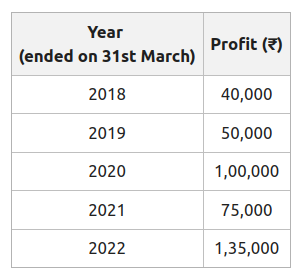2. Capital Employed is ₹2,50,000.

3.  Normal Rate of Return is 20%.

4. Average Profit is ₹80,000.

Solution:

Calculating Super Profit:

Normal Profit = 20% of Capital Employed i.e., 20% of 2,50,000 = ₹50,000

Super Profit = Average Profit – Normal Profit

Super Profit = 80,000 − 50,000

Super Profit = ₹30,000

Calculation of Firm’s Goodwill:

Goodwill =Goodwill =Goodwill = ₹1,50,000

My Personal Notes arrow_drop_up
Related Articles